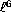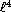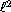# waynehu

## Professor, Department of Astronomy and Astrophysics University of Chicago

Group Contact CV SnapShots

## Scalars, Vectors, & Tensors

There are three types of fluctuations: scalars, vectors and tensors, and four observables: the temperature, E-mode, B-mode, and temperature cross polarization power spectra. The CMB thus provides sufficient information to separate these contributions, which in turn can tell us about the generation mechanism for fluctuations in the early universe (see §4.5).

Ignoring for the moment the question of foregrounds, to which we turn in §5.2, if the E-mode polarization greatly exceeds the B-mode then scalar fluctuations dominate the anisotropy. Conversely if the B-mode is greater than the E-mode, then vectors dominate. If tensors dominate, then the E and B are comparable (see Fig. 15). These statements are independent of the dynamics and underlying spectrum of the perturbations themselves.

The causal constraint on the generation of a quadrupole moment (and hence the polarization) introduces further distinctions. It tells us that the polarization peaks around the scale the horizon subtends at last scattering. This is about a degree in a flat universe and scales with the angular diameter distance to last scattering. Geometric projection tells us that the low-tails of the polarization can fall no faster than,andfor scalars, vectors and tensors (see §3.2). The cross spectrum falls no more rapidly thanfor each. We shall see below that these are the predicted slopes of an isocurvature model.

Furthermore, causality sets the scale that separates the large and small angle temperature-polarization correlation pattern. Well above this scale, scalar and vector fluctuations should show anticorrelation (tangential around hot spots) whereas tensor perturbations should show correlations (radial around hot spots).

Of course one must use measurements at small enough angular scales that reionization is not a source of confusion and one must understand the contamination from foregrounds extremely well. The latter is especially true at large angles where the polarization amplitude decreases rapidly.

Fig. 15: Tensor power spectra. The tensor temperature, temperature-cross-polarization, E-mode and B-mode polarization for a scale invariant intial spectrum of tensors with &Omega0=1, &OmegaB=0.1, h=0.5. The normalization has been artificially set (high) to have the same quadrupole as the scalar spectrum of Fig. 14. Also shown is the MAP 1&sigma upper limits on the B-modes due to noise, i.e. assuming no signal, showing that higher sensitivity will likely be necessary to obtain a significant detection of B-polarization from tensors.